Courses

# Olympiad Test: Electricity & Circuits

## 20 Questions MCQ Test Science Class 6 | Olympiad Test: Electricity & Circuits

Description
This mock test of Olympiad Test: Electricity & Circuits for Class 6 helps you for every Class 6 entrance exam. This contains 20 Multiple Choice Questions for Class 6 Olympiad Test: Electricity & Circuits (mcq) to study with solutions a complete question bank. The solved questions answers in this Olympiad Test: Electricity & Circuits quiz give you a good mix of easy questions and tough questions. Class 6 students definitely take this Olympiad Test: Electricity & Circuits exercise for a better result in the exam. You can find other Olympiad Test: Electricity & Circuits extra questions, long questions & short questions for Class 6 on EduRev as well by searching above.
QUESTION: 1

### The diagram shows identical lamps X and Y connected in series with a battery. The lamps light with normal brightness.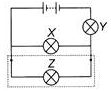If a third lamp Z is connected in parallel with lamp X, then what will happens to the brightness of the lamp V?

Solution:

The third lamp Z is connected parallel to X, so the current gets divided among X and Z. So both bulbs X and Z light dimmer and the bulb Y lights brighter than earlier because current through it increases.

QUESTION: 2

### Look at the given figure. It consists of a cell, a bulb with the two terminals X and Y and wires with ends P and 0; and S and R. The direction of current will be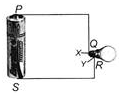Solution:

The direction of current will be PQRS because the current flows from positive terminal of the cell to the negative terminal

QUESTION: 3

### In which of the following circuits will the bulb glow?

Solution:

In figure (A), both ends of the bulb are connected to the negative terminal of the cell. So, bulb will not glow while in figure (B) and (C), insulating objects like string and rubber band are used. So, the circuit is incomplete and bulb will not glow. Figure (D) represents the complete circuit with proper connection. Hence, the bulb glows in figure (D).

QUESTION: 4

Which part of the bulb is an insulator?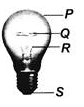Solution:

P is the glass cover, Q is the filament, R is the supporting wires, while S is the positive terminal of the bulb.

QUESTION: 5

To protect the user, part(s) ____ of the plug as shown in the figure must be made of electrical insulators.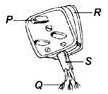Solution:

The plug cover R and connecting wires cover S should be made of electrical insulators.

QUESTION: 6

There is a mistake in the given classification table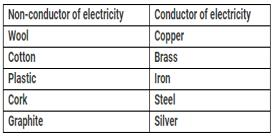Which of the following is placed incorrectly in the groups?

Solution:

Graphite is a conductor of electricity.

QUESTION: 7

The wire which carries away the current to the power house is called

Solution:

Reflection takes place whenever ray of light travels from a transparent medium towards an opaque medium . The opaque medium does not let the light pass through.

QUESTION: 8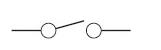What is the function of the component shown above?

Solution:
QUESTION: 9

Which of the following statements are correct?
(i) Human body is a good conductor of electricity.
(ii) The air gap surrounding an electric circuit acts as a conductor.
(iii) Silver conducts electricity better than any other metal.
(iv) When electricity lights up a bulb, no heat is produced.

Solution:

Air is a bad conductor of electricity. When electricity lights up a bulb, heat is produced.

QUESTION: 10

Study the classification carefully and select the correct option for X, Y and Z.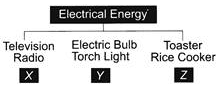Solution:

In X, the electrical energy is converted into sound energy. In Y, the electrical energy is converted into light energy. In Z, the electrical energy gets converted into heat energy. So, (C) is the correct option.

QUESTION: 11

"Do not handle electric wires with your bare hands. Wear rubber gloves," said Mr Gupta. Which of the following statements is not true about what Mr. Gupta commented?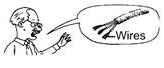Solution:

The rubber gloves are bad conductors of electricity. Hence, they prevent electric current to enter in our body and protect us from electric shocks.

QUESTION: 12

Nidhi has two bulbs connected across two cells in a simple circuit as shown. How can she make the bulbs glow dimmer?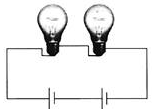Solution:

Replacing one cell with a piece of wire reduces the current in the circuit. Hence, the bulbs glow dimmer.

QUESTION: 13

Fuse is the most important safety device, used for protecting the circuits due to

Solution:

Fuse is a device used for short circuiting the circuit when overloading of current happens which helps to protect the electrical appliances.

QUESTION: 14

Direction: Observe the given figure and answer the following question.
The bulb will glow when a _____ is placed in between the probes.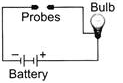Solution:

Key is a metallic object. When it is placed in between probes then current can easily flow in the circuit.

QUESTION: 15

Direction: Observe the given figure and answer the following question.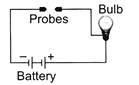Which of the following statements is correct regarding the maximum brightness of the bulb used in the circuit?

Solution:

Brightness of bulb can be increased by increasing the current through the bulb. This can be achieved by connecting a similar battery in the circuit.

QUESTION: 16

Three identical bulbs namely P, Q and R are connected to a battery as shown. What will happen when the circuit is closed?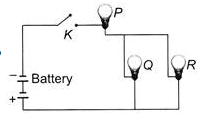Solution:

When the circuit is closed, an electric current starts flowing in the circuit from the positive terminal of battery. The current which passes through bulb P gets divided and flows in bulbs Q and R. As the brightness depends upon the current flowing through the bulb, so P will be bright but Q and R will be dim.

QUESTION: 17

In the given diagram, there are two switches S1 and S2 and two bulbs X and Y. If switch S1, is closed and switch S2 is open then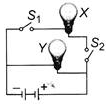Solution:

Since switch S2 is open and S1 is closed, the current will pass through Y but for bulb X, the circuit is incomplete. There is no complete path for current to flow for bulb X. Hence, only bulb Y will glow.

QUESTION: 18

Ravi connected three bulbs with the cells and a switch as shown. When switch is moved to ON position,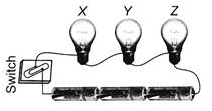Solution:

Current will flow positive to negative terminal, so all bulbs will glow simultaneously.

QUESTION: 19

Manu connected three bulbs to a cell as shown in figure. She found that filament of bulb is broken. Which of the following statements is correct?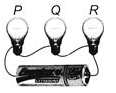Solution:

Bulb Q is fused because its filament is broken and as it is fused the circuit is broken. So none of the bulb will glow.

QUESTION: 20

Given diagram is a torch containing cells. The torch is not glowing when the switch is ON. Now, it is opened to know the reasons. Which of the following statements are correct regarding the reasons?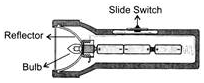(i) Bulb of the torch is fused.
(ii) Cells are not connected properly.
(iii) The metal parts of the torch do not make proper contact with the cell.

Solution:

The torch is not glowing because either its bulb is fused or cells are connected wrongly or metal spring of torch does not make proper contact with the cell.